## Solving Exercise 7: Properties of the three medians of a triangle (C8 Math 7 Horizons) – Math Book

Solution for Exercise 7: Properties of the three medians of a triangle (C8 Math 7 Horizons)
==========

### Solution 1 page 75 Math textbook 7 Creative horizon volume 2 – CTST

Observe Figure 8.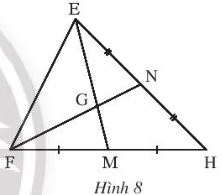Find the correct number to fill in the dot in the following equations:

$$\begin{array}{l}EG = …EM;\,\,\,GM = …EM;\,\,\,\,GM = …EG\\FG = …GN;\,\,\ ,\,\,FN = …GN;\,\,\,\,\,FN = …FG\end{array}$$

Detailed instructions for solving Lesson 1

Solution method

We rely on the theorem that three medians intersect at a point. That point is a distance from each vertex equal to $$\dfrac{2}{3}$$ the length of the median passing through that vertex.

Detailed explanation

$$\begin{array}{l}EG = \dfrac{2}{3}EM;\,\,\,GM = \dfrac{1}{3}EM;\,\,\,\,GM = \dfrac{1}{2}EG\\FG = 2GN;\,\,\,\,\,FN = 3GN;\,\,\,\,\,\,\,FN = \dfrac{3} {2}FG\end{array}$$

–>

— *****

### Solve problem 2 page 75 Math textbook 7 Creative horizon volume 2 – CTST

Observe Figure 9

a) Knowing AM = 15 cm, calculate AG

b) Knowing GN = 6 cm, calculate CN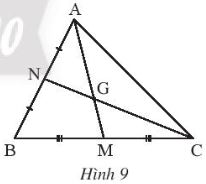Detailed instructions for solving Lesson 2

Solution method

– We rely on the theorem of three medians intersecting at 1 point. That point is a distance from each vertex equal to $$\dfrac{2}{3}$$the length of the median passing through that vertex.

– We apply the ratios between the lines and their lengths

Detailed explanation

a) According to the problem, we have AM = 15 cm

Where CN and AM are the two medians of triangle ABC

AM intersects CN at G, so G is the centroid of triangle ABC

$$\Rightarrow AG = \dfrac{2}{3}AM$$(center of triangle theorem)

$$\Rightarrow AG = \dfrac{2}{3}\,15cm = 10cm$$

b) Since G is the centroid of triangle ABC

$$\Rightarrow CG = \dfrac{2}{3}CN$$(according to the property of the median passing through the centroid)

And $$CG + GN = CN$$ so we have $$GN = CN – CG = CN – \dfrac{2}{3}CN = \dfrac{1}{3}CN$$

Under the assumption GN = 6cm we have

$$\Rightarrow CN = 3GN = 3.6cm = 18cm$$

–>

— *****

### Solution 3 page 75 Math textbook 7 Creative horizon volume 2 – CTST

Let ABC be a triangle. The two medians AM and CN intersect at G. On the opposite ray of the ray AM take the point E such that ME = MG.

a) Prove that BG is parallel to EC.

b) Let I be the midpoint of BE, AI intersects BG at F. Prove that AF = 2FI

Detailed instructions for solving Lesson 3

Solution method

– We rely on the theorem of three medians intersecting at 1 point. That point is a distance from each vertex equal to $$\dfrac{2}{3}$$the length of the median passing through that vertex.

– Sentence a will prove that 2 staggered interior angles are congruent through congruent triangles

– Sentence b will prove that F is the centroid of triangle ABE

Detailed explanation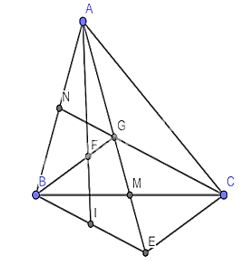a) Consider triangle BGM and triangle CEM:

$$\widehat {GMB} = \widehat {EMC}$$(2 opposite angles)

GM = ME (because G is symmetric about E through M)

MB = MC (since M is mid point of BC)

$$\Rightarrow \Delta BGM = \Delta CEM(c – g – c)$$

$$\Rightarrow \widehat {GBM} = \widehat {MCE}$$(2 corresponding angles are equal)

The two upper corners are in staggered positions, so BG⫽CE

b) Since I is the midpoint of BE, AI will be the median of triangle ABE

And BG is also the median of triangle ABE since G is the midpoint of AE

Since BG intersects AI at F, F will be the centroid of triangle ABE

$$\, \Rightarrow AF = \dfrac{2}{3}AI$$(triangle centroid theorem)

Which AI = AF + FI $$\Rightarrow$$ FI = AI – AF

$$\Rightarrow FI = AI – \dfrac{2}{3}AI = \dfrac{1}{3}AI$$

$$\Rightarrow 2FI = AF = \dfrac{2}{3}AI$$

$$\Rightarrow$$ AF = 2 FI

–>

— *****

### Solve problems 4 pages 75 Math textbook 7 Creative horizon volume 2 – CTST

Let ABC be an isosceles triangle at A with BM and CN as the two medians.

a) Prove that BM = CN

b) Let I be the intersection of BM and CN, the line AI intersects BC at H. Prove that H is the midpoint of BC.

Detailed instructions for solving Lesson 2

Solution method

– We prove that two triangles are congruent so that we can prove that the two segments are congruent

– We prove that I is the centroid of triangle ABC and prove that AH is the median of triangle ABC and H is the midpoint of BC.

Detailed explanation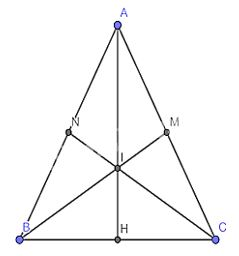a) Because triangle ABC is isosceles at A by assumption. BM and CN are 2 medians, so M and N are the midpoints of AC and AB.

Because AB = AC (isosceles triangle property)

$$\Rightarrow \dfrac{{AB}}{2} = \dfrac{{AC}}{2} = AN = AM$$

Considering triangle AMB and triangle ANC, we have:

AM = AN (cmt)

AB = AC

Common angle A

$$\Rightarrow \Delta AMB =\Delta ANC$$

$$\Rightarrow BM = CN$$ ( 2 corresponding edges )

b) Since BM and CN are medians

Where I is the intersection of BM and CN

$$\Rightarrow$$ I is the centroid of triangle ABC

$$\Rightarrow$$ AI is the median of triangle ABC or AH is the median of triangle ABC

$$\Rightarrow$$ H is the midpoint of BC

–>

— *****

### Solve problems 5 pages 76 Math textbook 7 Creative horizon volume 2 – CTST

Let ABC be a triangle with median BM equal to median CN. Prove that triangle ABC is isosceles.

Detailed instructions for solving Lesson 5

Solution method

We prove AB = AC by proving that the two triangles are congruent

Detailed explanation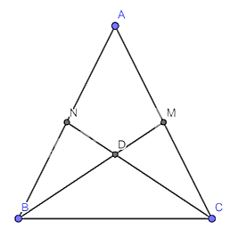Let D be the intersection of CN and BM .

$$\Rightarrow$$ D is the centroid of triangle ABC

$$\Rightarrow CD = \dfrac{2}{3}CN = BD = \dfrac{2}{3}BM$$ ( do BM = CN )

$$\Rightarrow$$ triangle DBC is isosceles at D since BD = CD

$$\Rightarrow$$ $$\widehat {DBC} = \widehat {DCB}$$(2 base angles in an isosceles triangle) (1)

Consider $$\Delta NDB$$ and $$\Delta MDC$$ with :

BD = CD

$$\widehat {NDB} = \widehat {MDC}$$ (2 opposite angles)

ND = DM (due to the same $$= \dfrac{1}{3}CN = \dfrac{1}{3}BM$$ (property of the orthocenter passing through the centroid of the triangle ))

$$\Rightarrow \Delta NDB=\Delta MDC$$ (cgc)

$$\Rightarrow \,\widehat {NBD} = \widehat {MCD}$$(2 corresponding angles) (2)

From (1) and (2) $$\Rightarrow \widehat {ABC} = \widehat {ACB}$$ do $$\widehat {ABC} = \widehat {NBD} + \widehat {DBC}$$ and $$\widehat {ACB} = \widehat {MCD} + \widehat {DCB}$$

$$\Rightarrow \Delta ABC$$ is equal at A (due to 2 equal angles)

–>

— *****

### Solve problem 6 page 76 Math textbook 7 Creative horizon volume 2 – CTST

Let ABC be an isosceles triangle at A with BD and CE being the two medians intersecting at F (Figure 10). Knowing BE = 9 cm, calculate the length of line segment DF.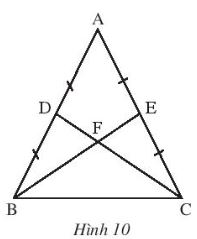Detailed instructions for solving Lesson 6

Solution method

– We prove that F is the centroid of triangle ABC

– Then prove CD = BE

– Applying the theorem about the center of gravity of the triangle, we calculate the segments DF, EF

Detailed explanation

Since BE and CD are the two medians of triangle ABC

SO E, D are medians of AB and AC . respectively

$$\Rightarrow AD = AE = \dfrac{1}{2}AB = \dfrac{1}{2}AC$$

Consider triangle ADC and triangle AEB:

Common angle A

AB = AC (triangle ABC is isosceles at A by assumption)

$$\Rightarrow \Delta ADC = \Delta AEB(c – g – c)$$

$$\Rightarrow BE = CD$$(corresponding edge)

Since F is the intersection of two medians, F is the centroid of triangle ABC

$$\Rightarrow CF = BF = \dfrac{2}{3}BE = \dfrac{2}{3}CD$$ ( theorem about median passing through centroid of triangle )

$$\Rightarrow \dfrac{1}{3}BE = \dfrac{1}{3}CD \Rightarrow DF = FE = \dfrac{1}{3}.9cm = 3cm$$

$$\Rightarrow$$ DF = 3 cm

–>

— *****